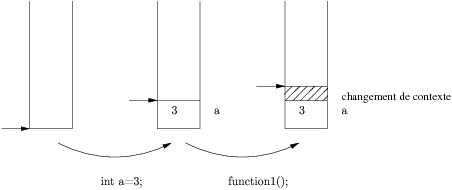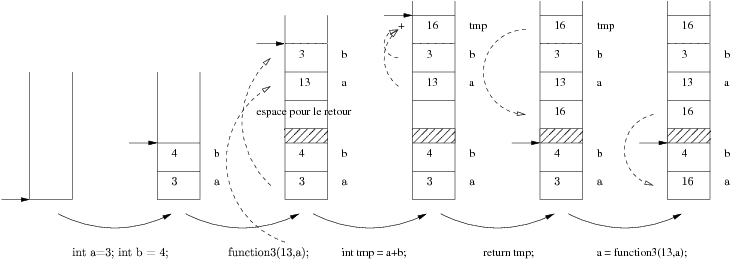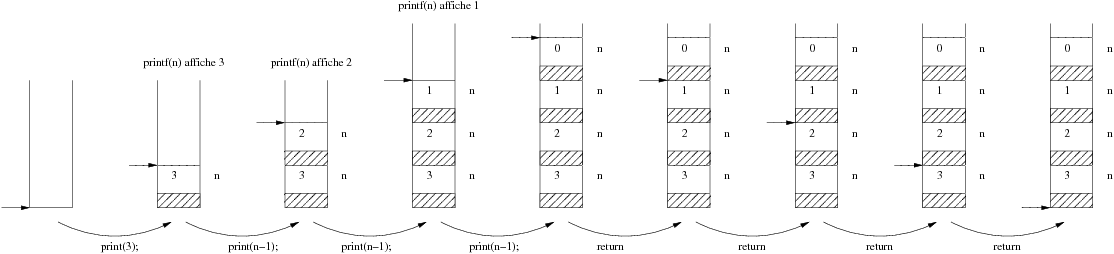### Functions

A C program code is organized into functions. A program always starts with the main() function. This function can, using an instruction, call the code written in an other function. Until the end of the execution of this function, the main is suspended. This can be done recursively (each function can call one or several other functions).

A function is a named block. The function call is different from a child block inside a parent block (see previous page) because the context of the calling function is no more accessible, ie the variables declared and define in the calling function are not accessible in the called function.

Example 1 :
`void function1(); int main(void){  int a = 3;  function1();  return 0;} void function1(){  printf("%d\n",a); /* NE MARCHE PAS ! */} `

In this example, the "a" variable, declared and defined in the main function, is not know in function1. However, it exists on the stack : (we do not represent what happens because of printf call)Remark: line 1 is a function declaration, and lines 9-11 are its definition.

Example 2 :
`void function2(); int main(void){  int a = 3;  function2();  printf("%d\n",a); /* affiche 3 */  return 0;} void function2(){  int a = 8;  printf("%d\n",a); /* affiche 8 */} `

Int this example, the "a" variable, declared and defined in the main, is still not known in function2. However, a other variable, existing in an other place in the stack, is also called a. The function can change his own variable a, it will not affect the "a" variable inside the main !Remark: at the end, the stack pointer is lower than its max value. This means that following variable declarations (in inner blocks or resulting from calling another functions) will overwrite the data use by the function.

### Parameters and return value

A function returning "void" cannot have side effect (except with pointers copy, see below).

Functions communicate with each others by two ways:

• The return value, which permits to a function to copy the content of one of its variable into a variable in the calling function. This return value determines the function type and the value the calling expression will takes (0 if void).
• Parameters: these are variables automatically declared (so added on the stack) when the function is entered. These variables are initialized with values that must be given during the function call.
• In both case, the values are copied between contexts. A function can only modify variable in there own contexts, except with pointers.

Example :
`void function3(); int main(void){  int a = 3;  int b = 4;  a = function3(13,a); /* la valeur de cette expression sera la valeur retournee */  return 0;} int function3(int a, int b){  int tmp = a+b;  return tmp;} `It is the compiler that check the types compatibility between the declaration and the call of the function.

### Recursive functions

A recursive function is a function that call itself. The behavior is the same as if it was calling another function. It is a powerful way to program, but use to consume more space on the stack.

Example : print all integer between 1 and n, n being a given parameter

`#include<stdio.h> void print(int n){  if(n>0){	  print(n-1); /* appel récurssif */	  printf("%d ",n);  }} int main(void){	print(3);	printf("\n");	return 0;} `

let look at what happens on the stack:And if we want to print from n to 1, easy: we just have to print the number before the recursive call:

`#include<stdio.h> void print_reverse(int n){  if(n>0){	  printf("%d ",n);	  print(n-1); /* appel récurssif */  }} int main(void){	print_reverse(3);	printf("\n");	return 0;} `

Let look at the stack: